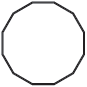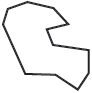# Dodecagon

A dodecagon is a polygon with 12 sides and 12 vertices. "Do-" means "two" and "deca-" means "ten."

## Dodecagon classifications

Like other polygons, a dodecagon can be classified as regular or irregular.

Regular dodecagonIrregular dodecagonAll sides and interior angles are congruentSides and angles have various lengths

A dodecagon can also be classified as convex or concave.

A convex dodecagon is a polygon where no line segment between any two points on its boundary lies outside of it. None of the interior angles is greater than 180°. Think of a convex dodecagon as bulging outwards, such as the regular dodecagon pictured above.

Conversely, a concave dodecagon, like the irregular dodecagon shown above, has at least one line segment that can be drawn between points on its boundary but lies outside of it. Also, at least one of its interior angles is greater than 180°.

A convex dodecagon does not have to be a regular dodecagon. Yet, a regular dodecagon is always a convex dodecagon. The dodecagon below is convex but clearly has sides and angles that are not congruent. This dodecagon is irregular.

## Angles of a dodecagon

Dodecagons can be broken into a series of triangles by diagonals drawn from its vertices. This series of triangles can be used to find the sum of the interior angles of the dodecagon.

Diagonals are drawn from vertex A in the convex dodecagon below, forming 10 triangles. Similarly, 10 triangles can also be drawn in a concave dodecagon. Since the sum of the degrees in a triangle is 180°, the sum of the interior angles of a dodecagon is 10 × 180° = 1800°.

A regular dodecagon has equal interior angle measures. Since 1800°/12 = 150°, each interior angle in a regular dodecagon has a measure of 150°. Also, each exterior angle has a measure of 30° since the exterior angle and interior angle form a straight angle.